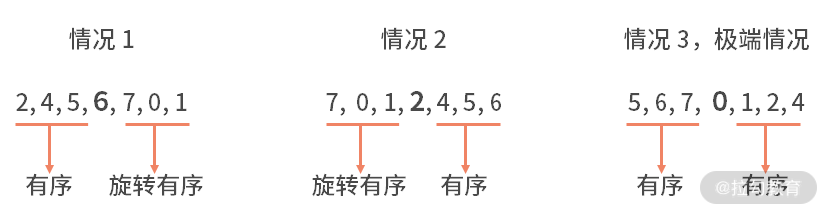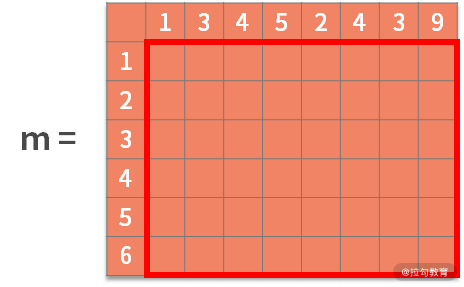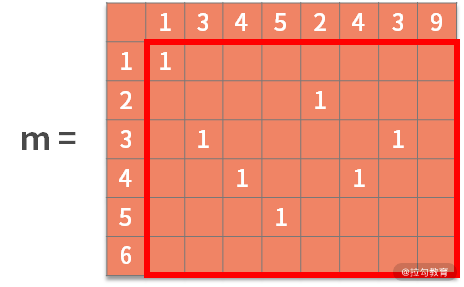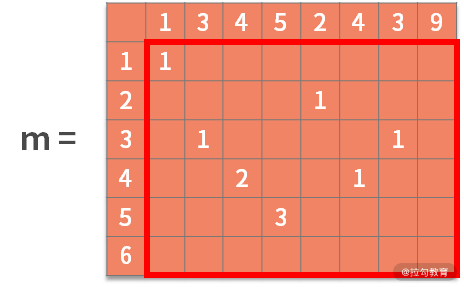16 真题案例（一）：算法思维训练

1. 复杂度分析。估算问题中复杂度的上限和下限。
2. 定位问题。根据问题类型，确定采用何种算法思维。
3. 数据操作分析。根据增、删、查和数据顺序关系去选择合适的数据结构，利用空间换取时间。
4. 编码实现

算法思维训练题

例题 1：斐波那契数列

【题目】写一个函数，输入 x，输出斐波那契数列中第 x 位的元素。例如，输入 4，输出 2；输入 9，输出 21。要求：需要用递归的方式来实现。

【解析】 在本课时开头，我们复习了解决代码问题的方法论，下面我们按照解题步骤进行详细分析。

• 首先我们还是先做好复杂度的分析

• 至于问题定位

• 数据操作方面

• 最后，实现代码

public static void main(String[] args) {
int x = 20;
System.out.println(fun(x));
}
private static int fun(int n) {
if (n == 1) {
return 0;
}
if (n == 2) {
return 1;
}
return fun(n - 1) + fun(n - 2);
}

• 递归体是第 13 行。即当输入变量 n 比 2 大的时候，递归地调用 fun() 函数，并传入 n-1 和 n-2，即 return fun(n - 1) + fun(n - 2)；
• 终止条件则是在第 7 行到第 12 行，分别定义了当 n 为 1 或 2 的时候，直接返回 0 或 1。

例题2：判断一个数组中是否存在某个数

【题目】给定一个经过任意位数的旋转后的排序数组，判断某个数是否在里面

【解析】 这道题目依旧是按照解决代码问题的方法论的步骤进行分析。

• 先做复杂度分析

• 接着，进入定位问题的环节中

• 然后，我们来做数据操作分析• 最后，实现代码

public static void main(String[] args) {
int[] arr = { 4, 5, 6, 7, 0, 1, 2 };
int target = 7;
System.out.println(bs(arr, target, 0, arr.length-1));
}
private static int bs(int[] arr, int target, int begin, int end) {
if (begin == end) {
if (target == arr[begin]){
return begin;
}
else{
return -1;
}
}
int middle = (begin + end)/2;
if (target == arr[middle]) {
return middle;
}
if (arr[begin] <= arr[middle-1]){
if (arr[begin] <= target && target <= arr[middle-1]) {
return bs(arr,target, begin,middle-1);
} else {
return bs(arr,target, middle+1,end);
}
}
else {
if (arr[middle+1] <= target && target <= arr[end]) {
return bs(arr,target, middle+1,end);
} else {
return bs(arr,target, begin,middle-1);
}
}
}

• 16 行计算分裂点的索引值。17 到 19 行，进行目标值与分裂点的判断。
• 如果相等，则查找到结果并返回；
• 如果不等就要继续二分。
• 在二分的过程中，第 20 行进行了左右子数组哪边是有序的判断。
• 如果左边有序，则进入到 21 到 25 行；
• 如果右边有序，则进入到 28 到 32 行。
• 假设左边有序，则还需要判断 target 是否在有序区间内，这是在第 21 行。
• 如果在，则继续递归的调用 bs(arr,target, begin,middle-1)；
• 如果不在有序部分，则说明 target 在另一边的旋转有序中，则调用 bs(arr,target, middle+1,end)。

• 在这里，需要判断最后剩下的 1 个元素是否与 target 相等：
• 如果相等则返回索引值；
• 如果不等则返回 -1。

例题3：求解最大公共子串

【题目】输入两个字符串，用动态规划的方法，求解出最大公共子串

【解析】这里已经定义了问题，就是寻找最大公共子串。同时也定义了方法，就是要用动态规划的方法。那么我们也不需要做太多的分析，只要依赖动态规划的步骤完成就可以了。

• 对于一个可能的起点，它后面的每个字符都是一个阶段。
• 状态就是当前寻找到的相匹配的字符。
• 决策就是当前找到的字符是否相等（相等则进入到公共子串中）。
• 状态转移方程可以写作 sk+1 = uk(sk)。可以理解为，如果 sk = "123"是公共子串，且在 a 字符串和 b 字符串中，"123"后面的字符相等，假设为"4"，则决策要进入到公共子串中，sk+1 = "1234"。
• 目标自然就是公共子串最长。
• 终止条件就是决策到了不相等的结果。public static void main(String[] args) {
String a = "13452439";
String b = "123456";
getCommenStr(a, b);
}
public static void getCommenStr(String a, String b) {
char[] c1 = a.toCharArray();
char[] c2 = b.toCharArray();
int[][] m = new int[c2.length+1][c1.length+1];
for (int i = 1; i <= c2.length; i++) {
for (int j = 1; j <= c1.length; j++) {
if (c2[i - 1] == c1[j - 1])
m[i][j] = m[i - 1][j - 1] + 1;
}
}
int max = 0;
int index = 0;
for (int i = 0; i <= c2.length; i++) {
for (int j = 0; j <= c1.length; j++) {
if (m[i][j] > max) {
max = m[i][j];
index = i;
}
}
}
String s = "";
for (int i = index - max; i < index; i++)
s += b.charAt(i);
System.out.println(s);
}